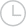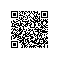# java读取excel内容-问答-阿里云开发者社区-阿里云

## java读取excel内容爵霸2016-03-23 15:18:392001

``````package data;
import java.io.File;
import jxl.*;
public class excelData {

public static void main(String[] args) {
int i;
Sheet sheet;
Workbook book;
Cell cell1,cell2,cell3;
try {
//t.xls为要读取的excel文件名
book= Workbook.getWorkbook(new File("D:\\java\\workspace\\DataAnalysis\\t.xls"));
/*
//获得第一个工作表对象(ecxel中sheet的编号从0开始,0,1,2,3,....)
sheet=book.getSheet(0);
//获取左上角的单元格
cell1=sheet.getCell(0,0);
System.out.println("标题："+cell1.getContents());

i=1;
while(true)
{
//获取每一行的单元格
cell1=sheet.getCell(0,i);//（列，行）
cell2=sheet.getCell(1,i);
cell3=sheet.getCell(2,i);
if("".equals(cell1.getContents())==true)    //如果读取的数据为空
break;
System.out.println(cell1.getContents()+"\t"+cell2.getContents()+"\t"+cell3.getContents());
i++;
}
book.close(); */
}
catch(Exception e)  { }

}

}``````取消 提交回答使用钉钉扫一扫加入圈子
+ 订阅